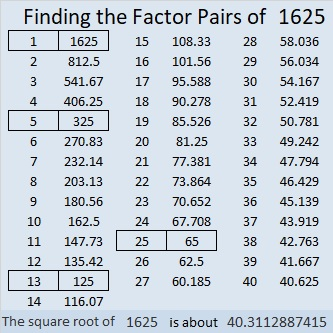# 1625 is a Centered Square Number

### Today’s Puzzle:

Because 1625 is the 29th centered square number, it is one more than four times the 28th triangular number. Can you draw lines on the graphic below separating out one tiny square and dividing the rest of the graphic into four equal triangles each with a base of 28 tiny squares?### Factors of 1625:

• 1625 is a composite number.
• Prime factorization: 1625 = 5 × 5 × 5 × 13, which can be written 1625 = 5³ × 13
• 1625 has at least one exponent greater than 1 in its prime factorization so √1625 can be simplified. Taking the factor pair from the factor pair table below with the largest square number factor, we get √1625 = (√25)(√65) = 5√
• The exponents in the prime factorization are 3 and 1. Adding one to each exponent and multiplying we get (3 + 1)(1 + 1) = 4 × 2 = 8. Therefore 1625 has exactly 8 factors.
• The factors of 1625 are outlined with their factor pairs in the graphic below.### More about the Number 1625:

1625 is the sum of two squares FOUR different ways:
40² + 5² = 1625,
37² + 16² = 1625,
35² + 20² = 1625, and
29² + 28² = 1625.

1625 is the hypotenuse of TEN Pythagorean triples:
57-1624-1625, calculated from 29² – 28², 2(29)(28), 29² + 28²,
180-1615-1625, which is 5 times (36-323-325),
400-1575-1625, calculated from 2(40)(5), 40² – 5², 40² + 5²,
455-1560-1625, which is (7-24-25) times 65,
572-1521-1625, which is 13 times (44-117-125),
625-1500-1625, which is (5-12-13) times 125,
825-1400-1625, calculated from 35² – 20², 2(35)(20), 35² + 20²,
975-1300-1625, which is (3-4-5) times 325,
1020-1265-1625, which is 5 times (204-253-325), and
1113-1184-1625, calculated from 37² – 16², 2(37)(16), 37² + 16².